Libri di Michele Zenga - libri Statistica & Applicazioni

# Michele Zenga

### Author's books

Editorial of the special issue on distribution and inequality measure in economics digital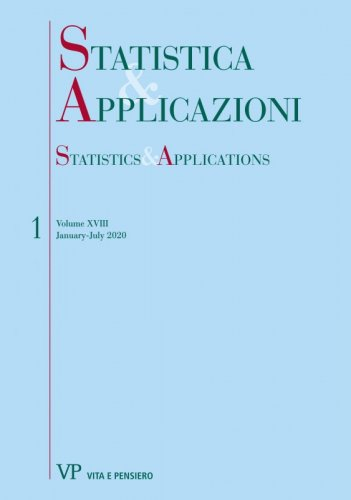format:Article| STATISTICA & APPLICAZIONI - 2020 - 1
Year: 2020
Decomposition by subpopulations of Gini, Bonferroni and Zenga inequality measures digitalformat:Article| STATISTICA & APPLICAZIONI - 2020 - 1
Year: 2020
This paper presents a common framework for the decompositions by subpopulations of Gini, Bonferroni and Zenga synthetic inequality measures. These three synthetic indexes are the weighted arithmetic means of the corresponding point measures and applying the Zenga two-step approach, decompositions based on means comparison are obtained. In the first step additive decompositions are derived for the point indexes and in the second step, using the decompositions of the point measures, we obtain the decompositions by subpopulations of the synthetic indexes...
Joint decomposition by subpopulations and sources of the point and synthetic Bonferroni inequality measures digital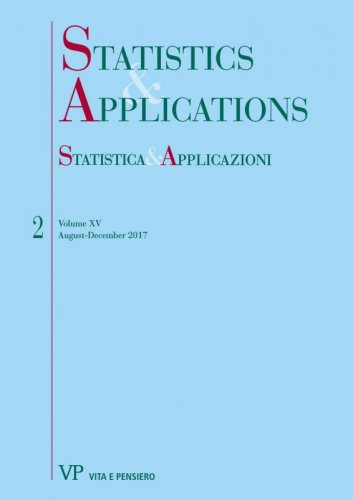format:Article| STATISTICA & APPLICAZIONI - 2017 - 2
Year: 2017
The total income Y is the sum of c sources Xj : Y = X1 + . . . + Xc: The N units of the population are partitioned in k different subpopulations. In the frequency distribution framework the Bonferroni (1930) point inequality index is given by Vh(Y) = [M(Y) - ̅Mh.(Y)]/M(Y), M(Y) and ̅Mh.(Y)are the mean and the lower mean of Y...
On the decomposition by subpopulations of the point and synthetic Bonferroni inequality measures digital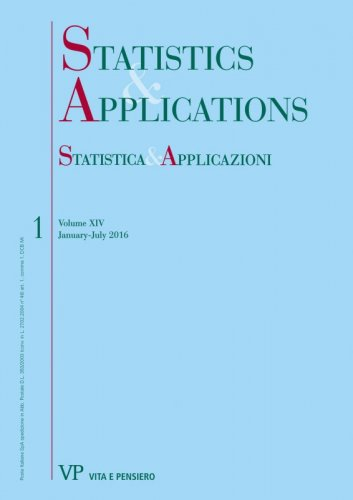format:Article| STATISTICA & APPLICAZIONI - 2016 - 1
Year: 2016
This paper, by using the ‘‘two-step’’ approach proposed in Radaelli (2008, 2010) and in Zenga (2016) for the decomposition of the Zenga (2007) index, obtains the decomposition of the Bonferroni (1930) inequality measure. In the first step the Bonferroni point measure Vh(Y) is decomposed in a weighted mean of k x k relative differences between the mean Mg(Y) of subpopulation g and the lower mean Mhl(Y) of the subpopulation l...
Decomposition by subpopulations of the point and the synthetic Gini inequality indexes digitalformat:Article| STATISTICA & APPLICAZIONI - 2016 - 1
Year: 2016
Keywords: Gini Index, Point Inequality Index, Synthetic Inequality Index, Decomposition by Subpopulatios
Joint decomposition by subpopulations and sources of the Zenga inequality index I(Y) digital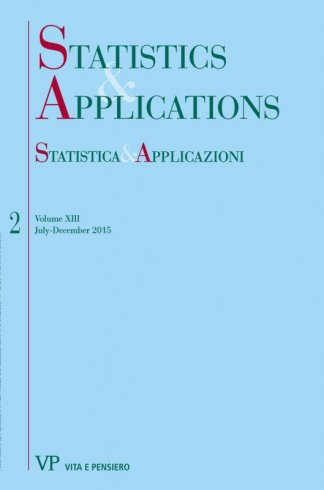format:Article| STATISTICA & APPLICAZIONI - 2015 - 2
Year: 2015
Keywords: Zenga Inequality Index, Income Inequality, Joint Decomposition by Subpopulations and Sources, Point and Synthetic Inequality Indexes.
The reordering variates in the decomposition by sources of inequality indexes digital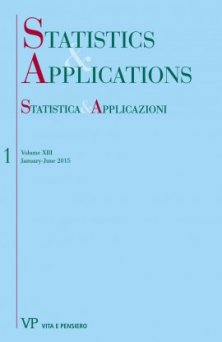format:Article| STATISTICA & APPLICAZIONI - 2015 - 1
Year: 2015
Keywords: Reordering Variate, Income Inequality, Decomposition by Sources, Point Inequality, Uniform Cograduation
Reasons for a translation
Free
digital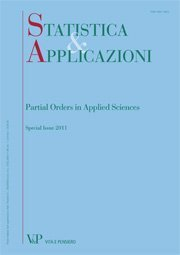format:Article| STATISTICA & APPLICAZIONI - 2013 - 1
Year: 2013
A longitudinal decomposition of Zenga’s new inequality Index
Free
digitalformat:Article| STATISTICA & APPLICAZIONI - 2013 - 1
Year: 2013
The paper proposes a three-term decomposition of Zenga’s new inequality index over time. Given an initial and a final time, the link among inequality trend, re-ranking, and income growth is explained by decomposing the inequality index at the final time into three components: one measuring the effect of re-ranking between individuals, a second term gauging the effect of disproportional growth between individuals’ incomes, and a third component measuring the impact of the inequality existing at the initial time. The decomposition allows one to distinguish the determinants of inequality change from the contribution of the inequality at the initial time to the inequality at the final time. We applied the decomposition to Italian household income data collected by the Survey on Household Income and Wealth of the Bank of Italy, waves 2008-2010.
Application of Zenga’s distribution to a panel survey on household incomes of European Member States
Free
digitalformat:Article| STATISTICA & APPLICAZIONI - 2013 - 1
Year: 2013
In this paper Zenga’s distribution is applied to 114 household incomes distributions from a panel survey conducted by Eurostat. Previous works showed the good behaviour of the model to describe income distributions and analyzed the possibility to impose restrictions on the parametric space so that the fitted models comply with some characteristics of interest of the samples. This work is the first application of the model on a wide number of distributions, showing that it can be used to describe incomes distributions of several countries. Maximum likelihood method on grouped data and methods based on the minimization of three different goodness of fit indexes are used to estimate parameters. The restriction that imposes the equivalence between the sample mean and the expected value of the fitted model is also considered. It results that the restriction should be used and the changes in fitting are analyzed in order to suggest which estimation method use jointly to the restriction. A final section is devoted to the direct proof that Zenga’s distribution has Paretian right-tail.
First applications of a new three-parameter distribution for non-negative variables
Free
digitalformat:Article| STATISTICA & APPLICAZIONI - 2012 - 2
Year: 2012
SUMMARY Zenga (2010a) recently proposed a new three-parameter family of density functions for non-negative variables. Its properties resemble those of economic size distributions: it has positive asymmetry, Paretian right tail and it may be zeromodal, unimodal or even bimodal. In this paper we explore some methods for fitting the new density to empirical income distributions. We will see that D’Addario’s invariants method clearly outperforms Pearson’s moments method, which does not seem to work well with heavy tailed distributions. Further, we propose some new methods based on the minimization of a measure for the goodness of fit, imposing restrictions on the parameter space to preserve some features of the empirical distribution in the fitted model. We will see that these methods provide very satisfactory results with income distributions from Italy, Swiss, US and UK. Keywords: Income Distribution, Zenga’s Distribution, Goodness of Fit, Moments Method, Invariants Method.
Decomposition of Zenga’s inequality index by sources
Free
digitalformat:Article| STATISTICA & APPLICAZIONI - 2012 - 1
Year: 2012
Point and interval estimation for some financial performance measures
Free
digitalformat:Article| STATISTICA & APPLICAZIONI - 2011 - 2
Year: 2011
We study the estimators of three financial performance measures: the Sharpe Ratio, the Mean Difference Ratio and the Mean Absolute Deviation Ratio. The analysis is performed under two sets of assumptions. First, the case of i.i.d. Normal returns is considered. After that, relaxing the normality assumption, the case of i.i.d. returns is investigated. In both situations, we study the bias of the estimators and we propose their bias-corrected version. The exact and asymptotic distribution of the three estimators is derived under the assumption of i.i.d. Normal returns. Concerning the case of i.i.d. returns, the asymptotic distribution of the estimators is provided. The latter distributions are used to define exact or asymptotic confidence intervals for the three indices. Finally, we perform a simulation study in order to assess the efficiency of the bias corrected estimators, the coverage accuracy and the length of the asymptotic confidence intervals. Keywords: Financial Performance Measure, Sharpe Ratio, Mean Difference Ratio, Mean Absolute Deviation Ratio, Concentration Measures, Statistical Analysis of Financial Data.
More on M.M. Zenga’s new three-parameter distribution for nonnegative variables
Free
digital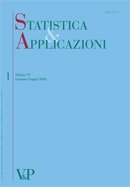format:Article| STATISTICA & APPLICAZIONI - 2011 - 1
Year: 2011
SUMMARY Recently Zenga (2010) has proposed a new three-parameter density function f (x : µ; α; θ), (µ > 0; α > 0; θ > 0), for non-negative variables. The parameter µ is equal to the expectation of the distribution. The new density has positive asymmetry and Paretian right tail. For θ > 1, Zenga (2010) has obtained the expressions of: the distribution function, the moments, the truncated moments, the mean deviation and Zenga’s (2007a) point inequality A(x) at x = µ. In the present paper, as to the general case θ > 0, the expressions of: the distribution function, the ordinary and truncated moments, the mean deviations and Zenga’s point inequality A (µ) are obtained. These expressions are more complex than those previously obtained for θ > 1 by Zenga (2010). The paper is enriched with many graphs of: the density functions (0.5 ≤ θ ≤ 1.5), the Lorenz L(p) and Zenga’s I (p) curves as well as the hazard and survival functions. Keywords: Non-Negative Variables, Positive Asymmetry, Paretian Right Tail, Beta Function, Lorenz Curve, Zenga’s Inequality Curve, Hazard Function, Survival Function.
Mixture of Polisicchio’s truncated Pareto distributions with beta weights
Free
digitalformat:Article| STATISTICA & APPLICAZIONI - 2010 - 1
Year: 2010
A new three-parameter density function f ðx : ; ; Þ for non-negative variables, obtained as a mixture of ‘‘Polisicchio’s truncated Pareto distributions’’, is proposed. The expectation of f ðx : ; ; Þ is equal to the parameter  > 0. The new density has positive asymmetry and Paretian right tail. The variance is equal to 2 3 ð þ 1Þ ½2 þ ð  1Þ   . The moments, the upper and lower truncated moments (with truncation at x ¼ ), are compact expressions of beta functions. Keywords: Income Distribution, Positive Asymmetry, Paretian Tail, Mixture Density, Beta Weights
Measuring loan recovery rate: methodology and empirical evidence
Free
digitalformat:Article| STATISTICA & APPLICAZIONI - 2008 - 2
Year: 2008
This paper aims at proposing a new methodology to compute recovery rate on non-performing bank loans, in order to confine this variable within the interval [0,1]. Such a methodology is then applied to data on loans gathered by the Bank of Italy and some interesting characteristics of the loan recovery process in the Italian banking market are highlighted. The combined effects of some variables on the recovery rates are also analysed. In particular, the presence of either collateral or personal guarantee, the borrower’s residence area are considered, thereby emphasizing the relationship between the recovery rate and the total exposure.
Asymmetry for ordinal variables
Free
digitalformat:Article| STATISTICA & APPLICAZIONI - 2007 - 2
Year: 2007
This paper proposes, for ordinal variables, an index of asymmetry based on the cumulative and retrocumulative frequencies. The paper shows that this new index has a connection with the bipolar mean, recently introduced by Maffenini and Zenga (2006). An application to a real case is presented to show how the asymmetry index works.
Inequality curve and inequality index based on the ratios between lower and upper arithmetic means
Free
digital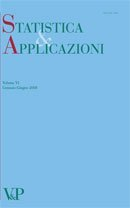format:Article| STATISTICA & APPLICAZIONI - 2007 - 1
Year: 2007
A new inequaliy curve I(p) based on the ratio between the lower mean M(p) and the upper mean þ M+(p) is proposed. By averaging I(p) the new inequality index I is obtained. The index I satisfies the usual properties required to an inequality measure. Being U(p)=1-I(p) a ratio between two arithmetic means, the meaning of I(p) is very straightforward. The index I is related to the Gini index R and the Bonferroni index B by the relation: R
Editorial digital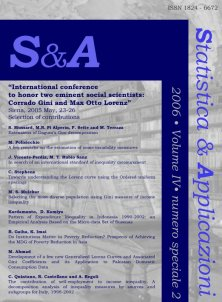format:Article| STATISTICA & APPLICAZIONI - 2006 - Special issue 2
Year: 2006
This second special issue of Statistica & Applicazioni provides a selection of papers, which were presented and discussed at the International Conference to Honor Two Eminent Social Scientists.
Convergence of the Sample Mean Difference to the normal distribution: simulation results digital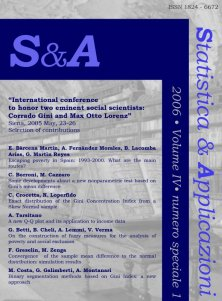format:Article| STATISTICA & APPLICAZIONI - 2006 - Special issue 1
Year: 2006
The present work aims to obtain the value of minimum sample size required by a good approximation by the normal curve for the sample mean difference. Particular care is given to what happens in the tails of the curves, with the aim of deriving confidence intervals for Gini’s mean difference. This goal is obtained by empirical methods and the presented results have an explorative nature. Simulation data have been obtained sampling from different distributions, considering symmetry versus asymmetry and the existence of the moments as main aspects in the underlying distribution. These remarks lead to the choice of the normal, the rectangular, the exponential and the Pareto distributions. All the obtained results indicate that the shape of the distribution from which the samples are generated is critically related to the minimum sample sizes required for a good approximation of the tails of the sample mean difference to the normal curve. Keywords: Gini Mean Difference, asymptotic distribution, convergence, U-statistic.
Editorial digitalformat:Article| STATISTICA & APPLICAZIONI - 2006 - Special issue 1
Year: 2006
This special issue of Statistica & Applicazioni provides a selection of papers, which were presented and discussed at the International Conference to Honor Two Eminent Social Scientists.
Bipolar mean for ordinal variables digital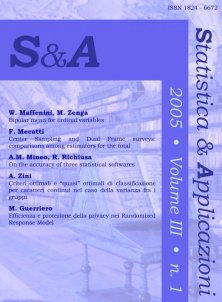format:Article| STATISTICA & APPLICAZIONI - 2005 - 1
Year: 2005
This paper proposes, for ordinal variables, a new type of mean called bipolar mean, that is a frequency distribution with the total size n concentrated on one category or on two consecutive categories.
On the Decomposition of the Gini’s Mean Difference and Concentration Ratio digital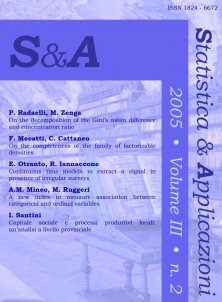format:Article| STATISTICA & APPLICAZIONI - 2005 - 2
Year: 2005
In this paper we propose a decomposition for the Gini’s mean difference of a real variate obtained as the sum of c variates observed on a finite population.
A partial ordering of dependence for contingency tables digital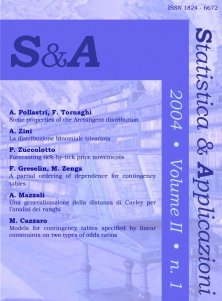format:Article| STATISTICA & APPLICAZIONI - 2004 - 1
Year: 2004
We introduce a new partial order according to the strength of dependence within the class of all contingency tables with given margins.
Distributive Compensation Ratio Derived from the Decomposition of the Mean Difference of a Sum digital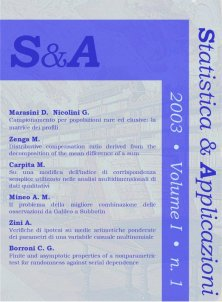format:Article| STATISTICA & APPLICAZIONI - 2003 - 1
Year: 2003
In this paper a distributive compensation index, based on the decomposition of the mean difference of a sum Y of the k variates X1, … , Xi, … , Xk , has been proposed.
Convergence of the Sample Mean Difference to the normal distribution: simulation results digitalformat:Article| STATISTICA & APPLICAZIONI - 2006 - Special issue 1
Year: 2006
The present work aims to obtain the value of minimum sample size required by a good approximation by the normal curve for the sample mean difference.

### Browse the archive

#### 2020 issues

This site or third-party tools used by this make use of cookies necessary for the operation and useful for the purposes outlined in the cookie policy.